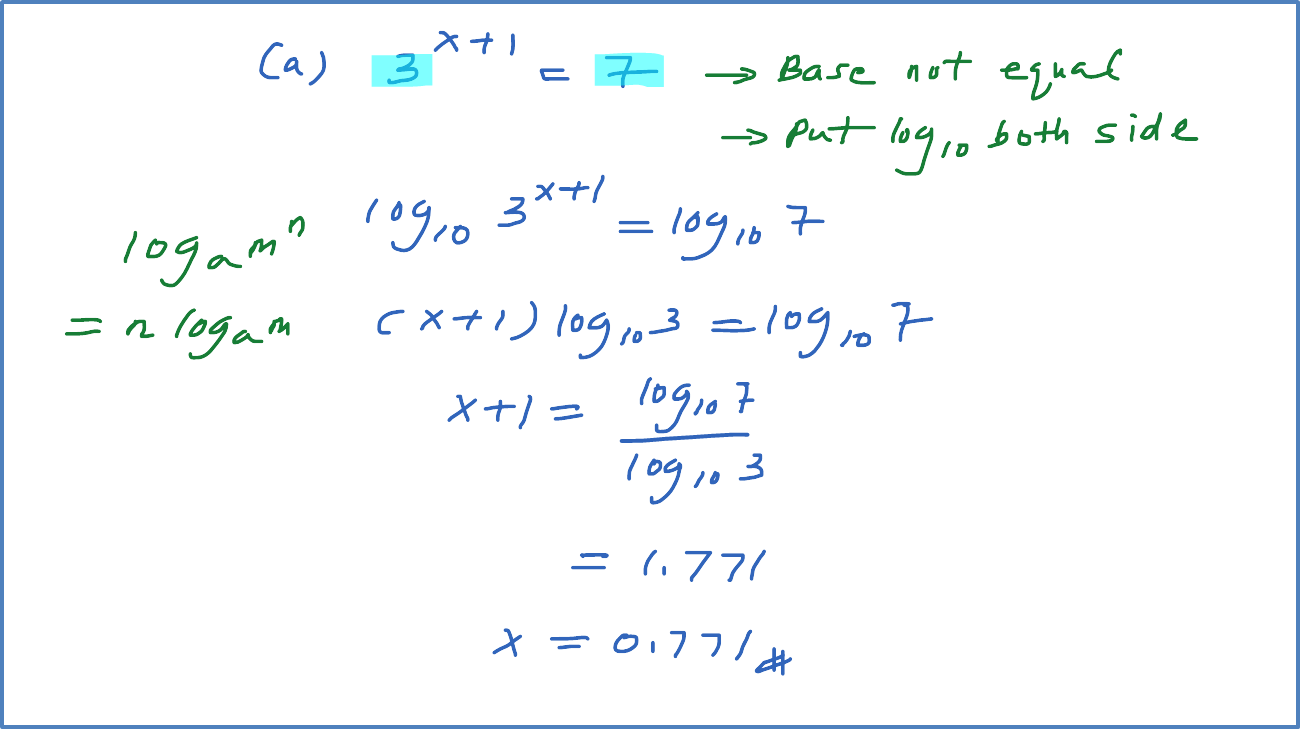# 5.3.5 Example 5 (Unequal Base – put log both side)

Example 5
Solve each of the following.
(a) ${3}^{x+1}=7$
(b) $2\left({3}^{x}\right)=5$
(c) ${2}^{x}{.3}^{x}={9}^{x-4}$
(d) ${5}^{x-1}{.3}^{x+2}=10$